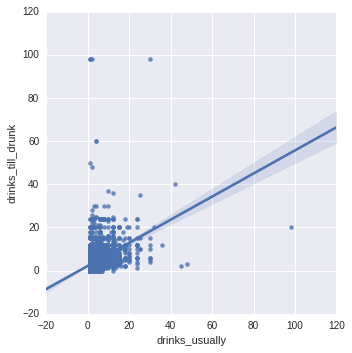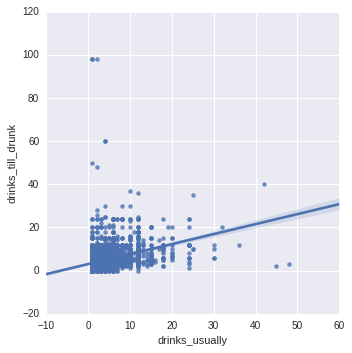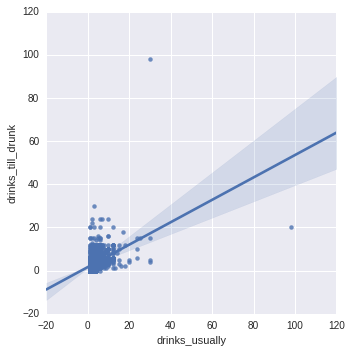# samucoder

Samuel M.H. 's technological blog

Notebook

# Statistical Interactions (Testing a Potential Moderator)¶

Author: Samuel M.H. <samuel.mh@gmail.com> Date: 31-01-2016

## Instructions

The final assignment deals with testing a potential moderator. When testing a potential moderator, we are asking the question whether there is an association between two constructs for different subgroups within the sample.

Run an ANOVA, Chi-Square Test or correlation coefficient that includes a moderator.

## What to submit:

Following completion of the steps described above, create a blog entry where you submit syntax used to test moderation (copied and pasted from your program) along with corresponding output and a few sentences of interpretation.

## Dataset

In :
%matplotlib inline

import pandas as pd
import matplotlib.pyplot as plt
import numpy as np
import scipy
import seaborn as sns


## Test of correlation

I am testing if there is a linear relationship (pearson correlation) between:

• Number of drinks of any alcohol usually consumed on days when drank alcohol in last 12 months.
• How many drinks can hold without feeling intoxicaded.

And if the sex is a moderator.

### Ingesting and curating the data

In :
#Load
#Select

df1 = pd.DataFrame()
df1['drinks_usually'] = pd.to_numeric(data['S2AQ8B'],errors='coerce').replace(99, np.nan)
df1['drinks_till_drunk'] = pd.to_numeric(data['S2AQ11'],errors='coerce').replace(99, np.nan)
df1['sex'] = data['SEX']
df1 = df1.dropna()
print(df1.shape)

(19740, 3)


In :
def corr(x,y,df):
r,p = scipy.stats.pearsonr(df[x],df[y])
print('Correlation coefficient (r): {0}'.format(r))
print('p-value: {0}'.format(p))
sns.lmplot(x=x, y=y, data=df)

In :
corr('drinks_usually','drinks_till_drunk',df1)

Correlation coefficient (r): 0.439569778654
p-value: 0.0There is medium (0,44) positive correlation with a p-value of 0, so it hasn't happened by chance.

## Moderator

Lets test if the sex is a moderator in the relationship.

In :
#Split data by sex
df_male = df1[(df1['sex']==1)]
df_female = df1[(df1['sex']==2)]


### Males

In :
corr('drinks_usually','drinks_till_drunk',df_male)

Correlation coefficient (r): 0.369791024379
p-value: 5.81135568843e-311### Females

In :
corr('drinks_usually','drinks_till_drunk',df_female)

Correlation coefficient (r): 0.484640967594
p-value: 0.0## Summary

Both results are significant but the variables are medium-weakly correlated (they don't really fit a linear model).

It is easily seen that the correlation is stronger in women when talking about alcohol tolerance.

So in this case, the sex is a moderator in the relationship between the number of drinks a person usually drinks and the number of drinks a person can take before feeling intoxicated because it affects the strength of the relationship.